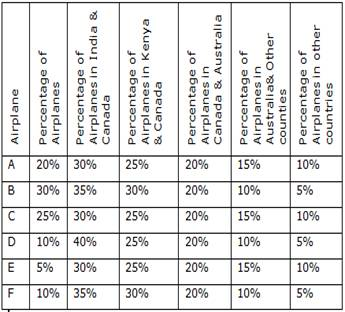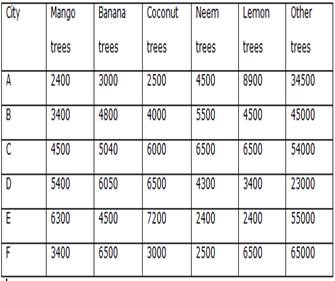# CRACK IBPS Clerk Prelims – Data Interpretation Day 24

Total number of Airplanes is 300000. Out of the total airplanes some airplanes are inaccessible.Q.1) what is the difference between the total number of airplanes in India and the total number of airplane in Kenya?

a) 16000

b) 24000

c) 18000

d) 20000

e) 22000

c

 Airplane Number of airplanes Number of airplanes in INDIA+FCANADA Number of airplanes in Kenya+CANADA Number of airplanes in CANADA+Australia Number of airplanes in Australia + other counties Number of airplanes in other countries A 60000 9000+9000 6000+9000 9000+3000 3000+6000 6000 B 90000 18000+13500 13500+13500 13500+4500 4500+4500 4500 C 75000 11250+11250 7500+11250 11250+3750 3750+7500 7500 D 30000 7500+4500 3000+4500 4500+1500 1500+1500 1500 E 15000 2250+2250 1500+2250 2250+750 750+1500 1500 F 30000 6000+4500 4500+4500 4500+1500 1500+1500 1500

Total number of airplanes in INDIA = 9000+18000+11250+7500+2250+6000 = 54000

Total number of airplanes in KENYA = 6000+13500+7500+3000+1500+4500 = 36000

Required difference = 54000-36000= 18000

Q.2) Number of airplane E in Kenya and Australia together is what percentage of the number of airplane F in INDIA?

a) 33.33%

b) 37.5%

c) 46.67%

d) 41.23%

e) 39.56%

b

 Airplane Number of airplanes Number of airplanes in INDIA+CANADA Number of airplanes in Kenya+CANADA Number of airplanes in CANADA+Australia Number of airplanes in Australia + other counties Number of airplanes in other countries A 60000 9000+9000 6000+9000 9000+3000 3000+6000 6000 B 90000 18000+13500 13500+13500 13500+4500 4500+4500 4500 C 75000 11250+11250 7500+11250 11250+3750 3750+7500 7500 D 30000 7500+4500 3000+4500 4500+1500 1500+1500 1500 E 15000 2250+2250 1500+2250 2250+750 750+1500 1500 F 30000 6000+4500 4500+4500 4500+1500 1500+1500 1500

Total number of airplane E in Kenya and Australia = 1500+750 = 2250

Number of airplane F in INDIA = 6000

Required % = 2250/6000×100=37.5%

Q.3) What is the ratio between the number of airplane C in Kenya and the number of airplane D in INDIA?

a) 1: 1

b) 3: 2

c) 2: 3

d) 4: 5

e) 7: 5

a

 Airplane Number of airplanes Number of airplanes in INDIA+CANADA Number of airplanes in Kenya+CANADA Number of airplanes in CANADA+Australia Number of airplanes in Australia + other counties Number of airplanes in other countries A 60000 9000+9000 6000+9000 9000+3000 3000+6000 6000 B 90000 18000+13500 13500+13500 13500+4500 4500+4500 4500 C 75000 11250+11250 7500+11250 11250+3750 3750+7500 7500 D 30000 7500+4500 3000+4500 4500+1500 1500+1500 1500 E 15000 2250+2250 1500+2250 2250+750 750+1500 1500 F 30000 6000+4500 4500+4500 4500+1500 1500+1500 1500

Number of airplane C in Kenya = 7500

Number of airplane D in INDIA = 7500

Required ratio = 1:1.

Q.4) what is the total number of airplanes in Canada?

a) 54000

b) 36000

c) 45000

d) 39000

e) 42000

c

 Airplane Number of airplanes Number of airplanes in INDIA+ Canada Number of airplanes in Kenya+ Canada Number of airplanes in Canada+ Australia Number of airplanes in Australia + other counties Number of airplanes in other countries A 60000 9000+9000 6000+9000 9000+3000 3000+6000 6000 B 90000 18000+13500 13500+13500 13500+4500 4500+4500 4500 C 75000 11250+11250 7500+11250 11250+3750 3750+7500 7500 D 30000 7500+4500 3000+4500 4500+1500 1500+1500 1500 E 15000 2250+2250 1500+2250 2250+750 750+1500 1500 F 30000 6000+4500 4500+4500 4500+1500 1500+1500 1500

Total number of airplanes in Canada = 9000+13500+11250+4500+2250+4500 = 45000

Q.5) The number of airplane B in INDIA and Australia together is what percentage more than the number of airplane C in same countries together?

a) 33.33%

b) 42%

c) 50%

d) 75%

e) None of these

c

 Airplane Number of airplanes Number of airplanes in INDIA+CANADA Number of airplanes in Kenya+CANADA Number of airplanes in CANADA+Australia Number of airplanes in Australia + other counties Number of airplanes in other countries A 60000 9000+9000 6000+9000 9000+3000 3000+6000 6000 B 90000 18000+13500 13500+13500 13500+4500 4500+4500 4500 C 75000 11250+11250 7500+11250 11250+3750 3750+7500 7500 D 30000 7500+4500 3000+4500 4500+1500 1500+1500 1500 E 15000 2250+2250 1500+2250 2250+750 750+1500 1500 F 30000 6000+4500 4500+4500 4500+1500 1500+1500 1500

The number of airplane B in INDIA  and Australia= 18000+4500= 22500

The number of airplane C in INDIA and Australia = 11250+3750 = 15000

Required % = 7500/15000×100=50%

The following table shows the number of different varieties of trees in various cities.Q.6) what is the average number of Lemon trees in various cities? (Approximately)

a) 5367

b) 5227

c) 5127

d) 5347

e) None of these

 City Mango trees Banana trees Coconut trees Neem trees Lemon trees Other trees Total A 2400 3000 2500 4500 8900 34500 55800 B 3400 4800 4000 5500 4500 45000 67200 C 4500 5040 6000 6500 6500 54000 82540 D 5400 6050 6500 4300 3400 23000 48650 E 6300 4500 7200 2400 2400 55000 77800 F 3400 6500 3000 2500 6500 65000 86900 Total 25400 29890 29200 25700 32200 276500

Required average = 32200/6 = 5366.67~5367

Q.7) what is the difference between the total number of trees in city C and the total number of trees  in city D?

a) 37780

b) 36780

c) 33890

d) 36390

e) 34780

c

 City Mango tree Banana tree Coconut tree Neem tree Lemon tree Other tree Total A 2400 3000 2500 4500 8900 34500 55800 B 3400 4800 4000 5500 4500 45000 67200 C 4500 5040 6000 6500 6500 54000 82540 D 5400 6050 6500 4300 3400 23000 48650 E 6300 4500 7200 2400 2400 55000 77800 F 3400 6500 3000 2500 6500 65000 86900 Total 25400 29890 29200 25700 32200 276500

Required difference= 82540-48650 = 33890

Q.8) The number of Coconut trees in the city E and city A together is what percentage of the total number of trees in the city B and city E together?

a) 4%

b) 5%

c) 7%

d) 5.5%

e) 9%

c

 City Mango tree Banana tree Coconut tree Neem tree Lemon tree Other tree Total A 2400 3000 2500 4500 8900 34500 55800 B 3400 4800 4000 5500 4500 45000 67200 C 4500 5040 6000 6500 6500 54000 82540 D 5400 6050 6500 4300 3400 23000 48650 E 6300 4500 7200 2400 2400 55000 77800 F 3400 6500 3000 2500 6500 65000 86900 Total 25400 29890 29200 25700 32200 276500

Required percentage = (2500+7200)/(67200+77800)×100= 7%

Q.9) What is the total number of trees in various cities?

a) 407890

b) 417890

c) 398920

d) 418890

e) None of these

d

 City Mango tree Banana tree Coconut tree Neem tree Lemon tree Other tree Total A 2400 3000 2500 4500 8900 34500 55800 B 3400 4800 4000 5500 4500 45000 67200 C 4500 5040 6000 6500 6500 54000 82540 D 5400 6050 6500 4300 3400 23000 48650 E 6300 4500 7200 2400 2400 55000 77800 F 3400 6500 3000 2500 6500 65000 86900 Total 25400 29890 29200 25700 32200 276500 418890

Required total = 418890

Q.10) If 10% of coconut trees in each city is destroyed, then what is the total number of remaining coconut trees in various cities?

a) 27380

b) 26880

c) 26480

d) 26180

e) 26280